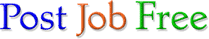# Python Data

Location:
San Leandro, CA
Posted:
September 08, 2018

#### Resume:

*

Yutai Shen, Ph.D.

San Leandro, CA ***** Tel: 310-***-**** Email: ac6yz3@r.postjobfree.com

* ***** ** ********** ** quantitative analysis and data science Skills

Machine Learning and Statistics: Regression Analysis, Neural Network, Cluster Analysis, PCA, Statistical hypothesis testing, Non-parametric Statistical inference, Time Series Analysis, Stochastic Process, Correlation analysis, Circular statistics.

Languages: Python (Numpy, Scipy, Matplotlib, Scikit-learn, Seaborn, Pandas, Nitime, PyCircStat, PyWavelets, Scikit-image), R (RStudio), SQL (MySQL), Octave/MATLAB, C.

Professional Experience

Shanxi Mount Heng Real Estate Development Limited, Taiyuan, China 03/2017 - present Remote Data Scientist

Performed data visualization, data preprocessing and descriptive statistics with Python modules Matplotlib, Pandas and Seaborn.

Trained unsupervised learning models of K-Means and clustered unlabeled data into groups based on semantic topic.

Implemented regression models with help of deep learning, employing PCA and ridge regression to address multicollinearity, utilizing Apache Spark, and Python modules TensorFlow and keras, to help the executive leadership in creating reasonable pricing strategies.

Trained stacked model combining Logistic Regression and Random Forest for prediction, with help of Python modules Pandas and Scikit-learn, to help evaluate consumers’ ability to pay. Department of Physics and Astronomy, UCLA. 11/2013 - 11/2016 Staff Research Associate 3 Neurophysics

Developed a resampling method which generated surrogate data by randomizing phase angles through Fourier Transform, utilizing Python Numpy.

Computed peak values, curvature and energy of waveforms from well identified spiking neurons, and constructed feature matrices, by dint of utilized Python module Numpy.

Computed PCA (a dimension reduction machine learning algorithm) for these feature matrices and trained a model by the first two principal components, with help of Python module Scikit-learn.

Applied this model and K-means (clustering algorithm of machine learning) through the Python Scikit-learn module to cluster neuronal spikes into excitatory or inhibitory groups.

Designed a novel denoising algorithm based on Fourier Transform, Inverse Fourier Transform curve fitting and Gaussian smoothing to remove the power line noise from the spectrum, with help of Python Scipy module and MATLAB.

Performed Time series analysis, including spectrum estimation, cross-spectrum estimation and time-frequency analyses, based on Fourier Transform with multitaper windowing functions, utilizing the MATLAB package chronux and Python Nitime.

Applied circular statistics with MATLAB circstat and Python module PyCircStat to analyze the phase preferences of discrete events relative to rhythmic activities.

Employed R (RStudio) and MATLAB to apply partial correlation to find the top influential behavior feature.

Implemented algorithms to detect individual transient ultrahigh frequency rhythmic pattern events from the continuous signal. The algorithms combined band-pass zeros-phase filtering, Hilbert Transform, utilizing the MATLAB and Python.

Applied Wavelet decomposition by dint of Python module PyWavelets and MATLAB Wavelet toolbox to analyze the distribution of peak frequencies of these detected transient ultrahigh frequency rhythmic pattern events.

Computed cross-correlation between of detected transient ultrahigh frequency rhythmic pattern events and acceleration, and employed resampling methods to estimate the confidential interval, with help of the Python and MATLAB. David Geffen School of Medicine, UCLA. 11/2012 - 10/2013 Postdoctoral Scholar Behavioral and Computational Neuroscience

Developed and optimized C codes to enable a proprietary experimental system. These C programs generated visual cue and stimulus with designated patterns and timing, recorded and evaluated the subjects’ reaction and eye movement traces, determined the experimental system’s feedback.

Designed and optimized an algorithm combining and employing binary file operation, spline interpolation, spectrum estimation, designing FIR filters and performing zeros-phase filtering to analyze eye movement signal, by MATLAB. State Key Laboratory of Cognitive Neuroscience and Learning, BNU. 09/2009 - 06/2012 PhD Student Cognitive Neuroscience

Designed and optimized algorithms to analyze neuronal population activities, combining auto-correlation, cross-correlation, spectrum and coherence, and several randomization resampling method, with help of the Python modules Scipy and Pandas.

Performed Time series analysis by utilizing continuous Gabor Transform to compute spectrogram, employing Python Scipy.

Utilized C programming to implement a custom-made experimental system. These C programs generated visual cue and stimulus with adjustable parameters to control task difficulty, recorded and evaluated the subjects’ behavior and determined the experimental system’s feedback accordingly, and communicated with the neuronal data collecting system. Education Experience

Beijing Normal University, Ph.D. in Cognitive Neuroscience Beijing, China 09/2009 - 06/2012

Shanxi Medical University, M.Sc. in Physiology Taiyuan, China 09/2006 - 06/2009

East China Normal University, B.Sc. in Biotechnology Shanghai, China 09/2001 - 06/2005

Contact this candidate